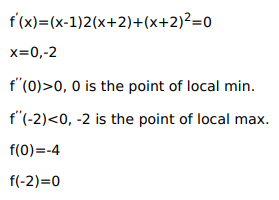# Find the point of local maxima or local minima or local minima and the corresponding

Question:

Find the point of local maxima or local minima or local minima and the corresponding local maximum and minimum values of each of the following functions:

$f(x)=(x-1)(x+2)^{2}$

Solution:

local max. value is 0 at $x=-2$ and local $\min$. value is $-4$ at $x=0$